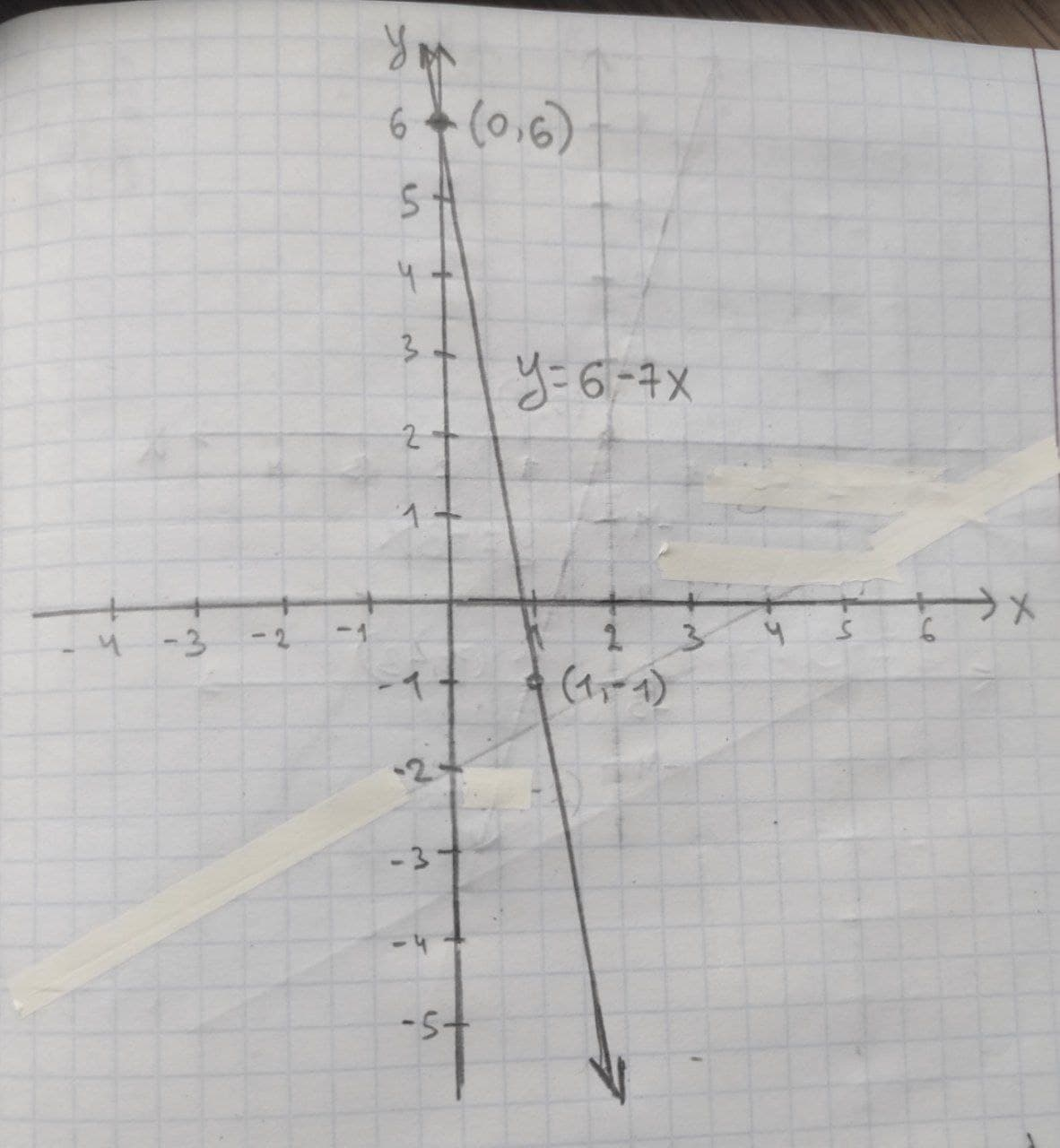Harlen Pritchard

2021-01-04

Given linear equation y = 6 − 7x
a. find the y-intercept and slope.
b. determine whether the line slopes upward, slopes downward, or is horizontal, without graphing the equation.
c. use two points to graph the equation.Arham Warner

(a) The linear equation is $y=6-7x$.
In a linear equation $y={b}_{0}+{b}_{1}x$ the constant ${b}_{1}$ be the slope and ${b}_{0}$ be the y-intercept form and $x$ is the independent variable and $y$ is the independent variable.
Comparing the given equation with the general form of linear equation the slope of the equation is –7 and the $y$-intercept is 6.
Thus, the slope of the linear equation is ${b}_{1}=-7$ and the $y$-intercept is ${b}_{0}=6$.
(b) In a linear equation $y={b}_{0}+{b}_{1}x$ the constant ${b}_{1}$be the slope and ${b}_{0}$ be the $y$-intercept form and $x$ is the independent variable and $y$ is the independent variable.
It is known that, the slope of the linear equation $y={b}_{0}+{b}_{1}x$ is upward if ${b}_{1}>0$, the slope of the linear equation $y={b}_{0}+{b}_{1}xy={b}_{0}+{b}_{1}x$ is downward if ${b}_{1}<0$ and the slope of the linear equation $y={b}_{0}+{b}_{1}x$ is horizontal if ${b}_{1}=0.$
Thus, in the given equation $y=6-7x,{b}_{1}=-7<0.$
Thus, the slope is downward.
(c) The linear equation is $y=6-7x$.
Graph the line:
The two points  on the given line are obtained:
If ,
$y=6+\left(-7×0\right)$
$y=6$
Thus, one point on the line is $\left({x}_{1}, {y}_{1}\right)=\left(0,6\right).$
If ,
$y=6+\left(-7×1\right)$
$y=-1$
Thus, the second point on the line is $\left({x}_{2}, {y}_{2}\right)=\left(1,-1\right).$Do you have a similar question?